Courses

# Test: Fluid Properties - 1

## 10 Questions MCQ Test Topicwise Question Bank for Civil Engineering | Test: Fluid Properties - 1

Description
This mock test of Test: Fluid Properties - 1 for Civil Engineering (CE) helps you for every Civil Engineering (CE) entrance exam. This contains 10 Multiple Choice Questions for Civil Engineering (CE) Test: Fluid Properties - 1 (mcq) to study with solutions a complete question bank. The solved questions answers in this Test: Fluid Properties - 1 quiz give you a good mix of easy questions and tough questions. Civil Engineering (CE) students definitely take this Test: Fluid Properties - 1 exercise for a better result in the exam. You can find other Test: Fluid Properties - 1 extra questions, long questions & short questions for Civil Engineering (CE) on EduRev as well by searching above.
QUESTION: 1

### If the dynamic viscosity of a fluid is 0.5 poise and specific gravity is 0.5, then kinematic viscosity of that fluid in stokes is

Solution:

μ = 0.05 Ns/m2
G = 0.5
∴  ρ = 0.5 x 1000 = 500 kg/m3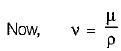∴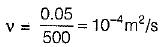= 1 cm2/s = 1 stokes

QUESTION: 2

### In a sample of water an increase of pressure by 18 MN/m2 caused 1% reduction in volume. The bulk modulus of elasticity of this sample, in MN/m2 is

Solution:

Bulk modulus of elasticity
k = Δp/(Δv/v) = 18/1/100
= 1800 MN/m2

QUESTION: 3

### The rise of liquid of specific weig ht ‘γ’ and  surface tension ‘σ’ in a capillary tube of radius ‘r’ is given by

Solution:

Height of capillary rise is given by,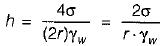QUESTION: 4

Work done in blowing a soap bubble of diameter 12 cm will be. Assume the surface tension of soap solution = 0.04 N/m.

Solution:

The soap bubble has two interfaces
∴ Work done
= 0.04 x 4π x (6 x 10-2)2 x 2
= 36.2 x 10-4 Nm

QUESTION: 5

The intensity of pressure developed by surface tension of 0.075 N/m in a droplet of water of 0.075 mm diameter is

Solution:

Pressure inside the liquid droplet is given by
Δp=4σ/d

σ is the surface tension = 0.075 N/m = (0.075/100) N/cm
d is the diameter = 0.075 mm= (0.075/10) cm

Therefore, pressure = [4 x (0.075/100)] / [0.075/10]
= 0.4 N/cm2

QUESTION: 6

The velocity distribution near the solid wall at a section in a laminar flow is given by u = 5 sin(5πy) for y < 0.1 m. The shear stress at a section y = 0.05m (if the dynamic viscosity of the fluid is 5 poise) will be

Solution: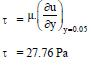QUESTION: 7

Surface tension of water

Solution:

In general, surface tension decreases when temperature increases because cohesive forces decrease with an increase of molecular thermal activity. The influence of the surrounding environment is due to the adhesive action of liquid molecules that they have at the interface.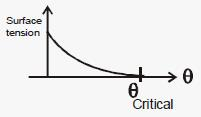QUESTION: 8

A Newtorian fluid fills the clearance between a shaft and a sleeve, when a force of 0.8 kN is applied to the shaft, parallel to the sleeve, the shaft attains a speed of 1.5 cm/s. If a force of 2.4 kN is applied instead the shaft would move with a speed of

Solution: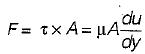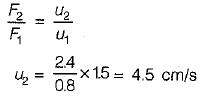QUESTION: 9

Consider the following statements:
1. Surface tension is due to cohesion only.
2. Capillarity is due to adhesion only.
3. Surface tension is due to both cohesion and adhesion.
4. Capillary is due to both cohesion and adhesion.

Which of these statements are correct?

Solution:
QUESTION: 10

If the relationship between the shear stress τ and the rate of shear strain du/dy is expressed as τ = k(du/dy)n then the fluid with a value of exponent n > 1 is known as

Solution: